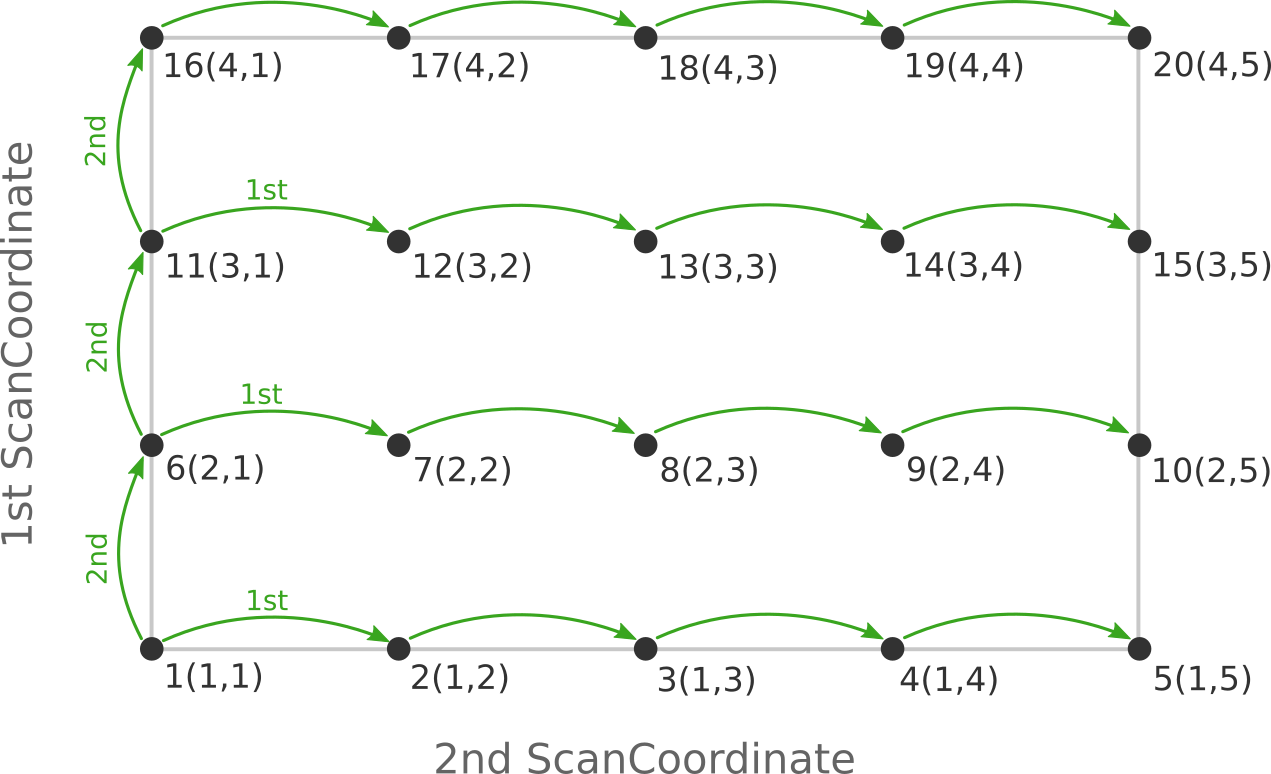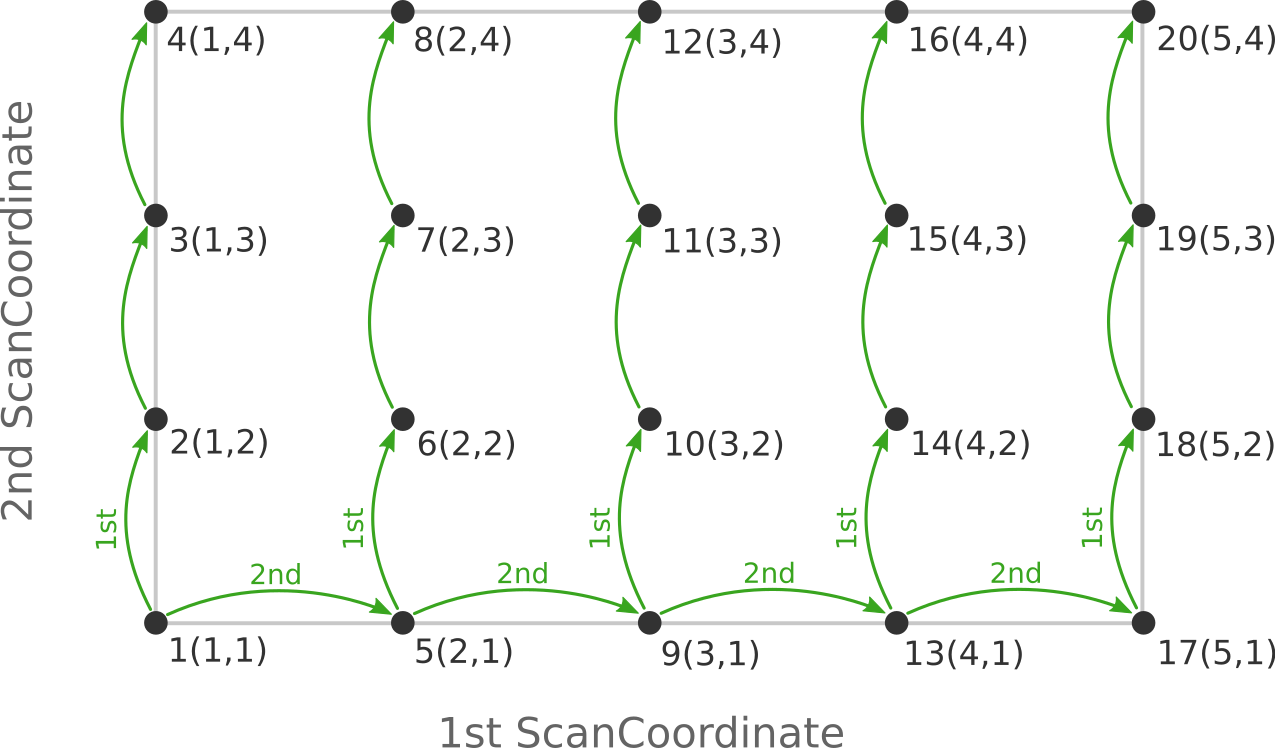# PES scan¶

The PES scan task in AMS allows users to scan the potential energy surface of a system along one or multiple degrees of freedom, while relaxing all other degrees of freedom. If only one coordinate is scanned, this kind of calculation is usually just called a linear transit. However, since AMS allows scanning of multiple coordinates, and linear transit is just a special case of such a calculation, the task is always called a PES scan in AMS.

The PES scan task is enabled by selecting it with the Task keyword:

Task PESScan


The PESScan block configures all details of the scan:

PESScan
CalcPropertiesAtPESPoints [True | False]
FillUnconvergedGaps [True | False]
ScanCoordinate
nPoints integer
Coordinate integer [x|y|z] (float){2}
Distance (integer){2} (float){2}
Angle (integer){3} (float){2}
Dihedral (integer){4} (float){2}
End
End


The PESScan block needs to contain at least one ScanCoordinate block specifying which coordinate to scan, and how many points (keyword nPoints) to sample along this coordinate. By default, 10 points are sampled along each scanned coordinate (including the start and end point of the scan). The coordinate descriptors are very similar to the constraint descriptors in the Constraints block used by the geometry optimization task, but are followed by two values delimiting the start and end of the coordinates, instead of just a single value:

Coordinate atomIdx [x|y|z] startValue endValue
Moves the atom with index atomIdx (following the order in the System block) along the a cartesian coordinate (x, y or z), starting at startValue and ending at endValue (given in Angstrom).
Distance atomIdx1 atomIdx2 startDist endDist
Scans the distance between the atoms with index atomIdx1 and atomIdx2, starting from startDist and ending at endDist, both given in Angstrom.
Angle atomIdx1 atomIdx2 atomIdx3 startAngle endAngle
Scans the angle (1)–(2)–(3) between the atoms with indices atomIdx1-3, as given by their order in the System%Atoms block. The scanned angle starts at startAngle and ends at endAngle, given in degrees.
Dihedral atomIdx1 atomIdx2 atomIdx3 atomIdx4 startAngle endAngle
Scans the dihedral angle (1)–(2)–(3)–(4) between the atoms with indices atomIdx1-4, as given by their order in the System%Atoms block. The scanned dihedral starts at startAngle and ends at endAngle, given in degrees.

Note that multiple of these coordinate descriptors can be combined within a single ScanCoordinate block. This combines the individual coordinates into one compound coordinate, i.e. all coordinates will transit together through their respective ranges. In this way the symmetric stretch in water could be scanned by specifying the following single ScanCoordinate block (assuming that the oxygen atom is the first in the System%Atoms block):

ScanCoordinate
Distance  1 2  0.8 1.1
Distance  1 3  0.8 1.1
End


A multidimensional PES scan can be performed by specifying multiple ScanCoordinate blocks in the input. To scan the space spanned by the bending and symmetric stretch modes in water, one would use the following scan coordinates:

ScanCoordinate
Distance  1 2  0.8 1.1
Distance  1 3  0.8 1.1
End
ScanCoordinate
Angle  2 1 3  90 130
End


In principle an arbitrary number of ScanCoordinate blocks can be combined to specify the scanned configuration space. However, the total number of sample points is the product of the number of points along all coordinates, and hence grows quickly with the number of dimensions. Furthermore, only 1D (linear transit) and 2D PES scans can be visualized in the GUI. We therefore suggest sticking with <=2 dimensional PES scans. (Note that it is possible to constrain additional degrees of freedom through the Constraints block. This could be used to sample a few points along a third dimension “manually”, while still being able to see the surfaces in the GUI.)

By default the engine result files for the individual PES points are not saved on disk, as this can easily lead to huge amounts of data to be stored. This behavior can be changed with the PESScan%CalcPropertiesAtPESPoints keyword:

PESScan
CalcPropertiesAtPESPoints
Type: Bool False Whether to perform an additional calculation with properties on all the sampled points of the PES. If this option is enabled AMS will produce a separate engine output file for every sampled PES point.

Note that this performs a full single point calculation on every sampled PES point, including the calculation of any PES point properties selected in Properties block.

## Troubleshooting¶

Technically all PES scan calculations are conducted as a series of geometry optimizations with constraints for the scanned coordinates, where the value of the constraint varies slowly through the scanned range. In this way every sampled point on the potential energy surface corresponds to a particular set of constraints. As with any geometry optimization, it can happen that an optimization towards a particular point on the potential energy surface does not converge. This is the most common problem encountered during PES scan calculations.

Since PES scans are implemented as a series of geometry optimizations, they are influenced by the settings used for the geometry optimizer, e.g. its convergence thresholds and the maximum number of steps before an optimization is considered to have failed. The optimizer is configured in the GeometryOptimization block, see the page on geometry optimization in the AMS manual. Note that PES scans always use the Quasi-Newton optimizer.

While tweaking the geometry optimizer’s settings can sometimes help with convergence problems, these problems can also be easily caused by errors in the user input.

A very common problem is that the geometry in the input, i.e. the System block, is incompatible with the starting values of the scanned coordinates. This would for example be the case if one wants to scan a dihedral angle from 0 to 90 degrees, but the actual angle on the input geometry is close to 90 degrees. In this case it would be better to flip the scanned range from 90 to 0 degrees, so that the input geometry already close to the first sampled point on the PES. Otherwise the optimization for the first point has to cross a very long distance on the PES, making convergence much harder. AMS automatically detects this and prints a warning. We generally advise preparing the input geometry for a PES scan by first running a geometry optimization with constraints set to lower bound of the scanned coordinate intervals.

For multidimensional PES scans the order in which the PES points are visited depends on the order in which the scanned coordinates are specified, i.e. the order of the ScanCoordinate blocks in the input. Generally, the order in which the PES points are visited is such that the coordinate which was specified in the first ScanCoordinate block varies slowest. This is illustrated in the following figure:Here the scan starts at point 1(1,1) at the bottom left corner of the PES and first moves along the entire range of the 2nd scan coordinate, before taking a step along the 1st coordinate to point 6(2,1). The same PES points could be visited in a different order (and under different names) if the order of the two ScanCoordinate blocks is reversed in the AMS input:Depending on the shape of the scanned potential energy surface a particular order of visiting the PES points might be easier or harder for the optimizer, and convergence problems can sometimes be fixed by simply changing the order of the scanned coordinates. In the example above, it might be that scanning along the “vertical” direction is “harder” than scanning along the “horizontal” direction. In this case one should use the scan order from the first picture, which has only three “vertical” steps (whereas the other scan order has 15).

Note that AMS has a little safe-guard built in to help with PES scan convergence issues: If the optimization towards a particular PES point did not succeed in the initial attempt, AMS will later try again, but starting from a different (converged) point close to unconverged one. This “PES gap filling” happens at the very end of the calculation, after the initial scan has been completed. This gap filling step is enabled by default, but can be controlled with the PESScan%FillUnconvergedGaps keyword:

PESScan
FillUnconvergedGaps
Type: Bool True After the initial pass over the PES, restart the unconverged points from converged neighboring points.